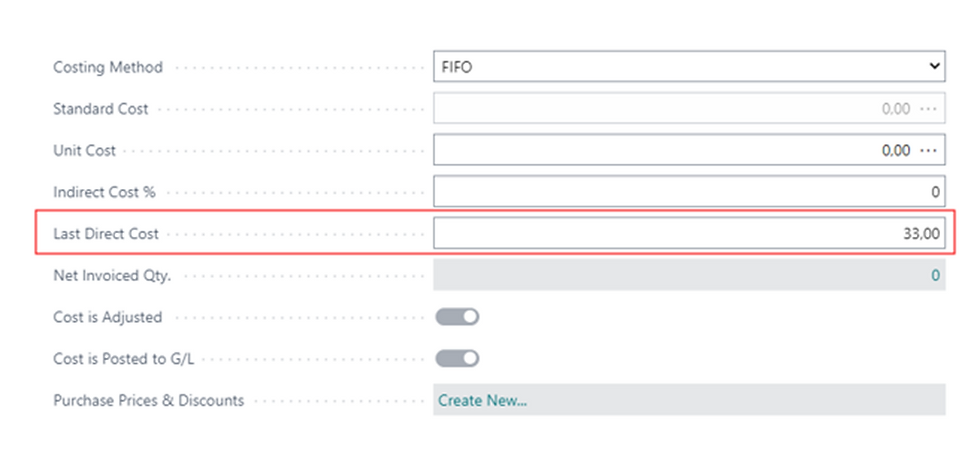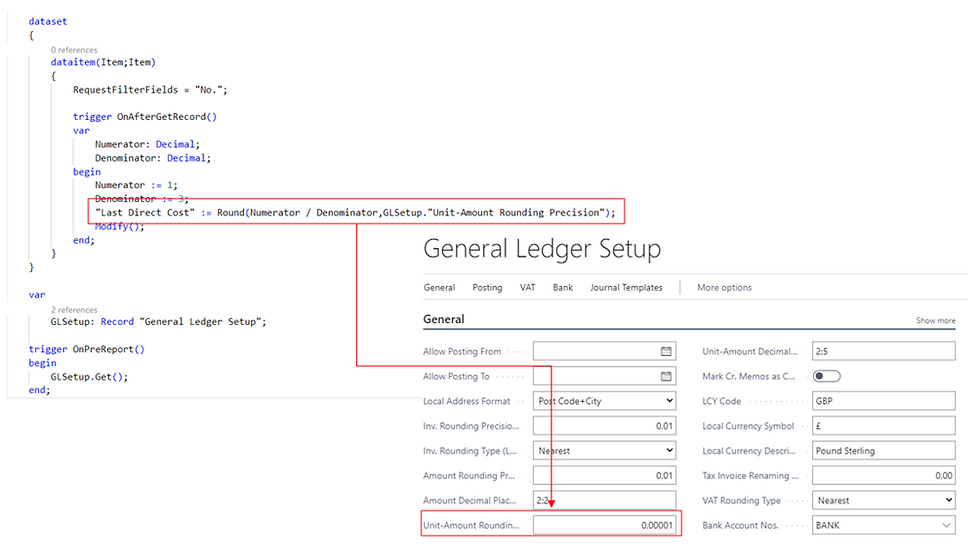top of page
Search
• marco ferrari

# DecimalPlaces, AutoFormatType and roudings: what we see and what the system does

We know that in Business Central we can enter formulas in each decimal field. This is a very useful feature that sometimes can avoid custom implementations for updating, for example, prices and costs. Suppose we need to increase the Last Direct Cost of an Item up to 10%. In order to do this, we just need to enter this formula into the fieldand get the final result when we move on another fieldNow the point is: what happens if we insert a formula resulting in a circulating decimal? The answer to this question is simply: the system will round the result to the maximum decimal places of the field.

In our specific case, the decimal numbers of the Last Direct Cost field are set by the AutoFormatType property, that dynamically sets the DecimalPlaces property to the value specified into the Unit-Amount Decimal Places (LCY) field of the General Ledger Setup tableSo, if we insert for example this formula into our fieldthe final result will beAs we know, what we see on a page does not necessarily correspond to the real content of the field, so we can make a check by opening the Page Inspection (CTRL+ALT+F1)Now suppose to create a batch that updates the field in this wayWhat happens? By opening the Item Card, we will see the same result as beforeBut by opening the Page Inspection (CTRL+ALT+F1), we will see a different numberBased on these results we can understand that working with numbers in Business Central can be subtle, especially if we work on prices and costs, because in that case each country is subject to a law that establishes how many decimal numbers we can manage on amounts/unit amounts for out currency and how to do roundings in calculations.

All these things are specified in the General Ledger Setup table, so our batch should be corrected in this way in order to get the correct result: